# Introduction

This is a short introduction and test for graphical models with stability selection. We first load the relevant packages. We use package QUIC to fit graphical networks:

``````library(stabs)
library(huge) # Need package huge for generating test data
``````
``````## Warning: package 'huge' was built under R version 3.4.1
``````
``````library(QUIC)
library(igraph)
``````

## Generate some test data using huge

``````N <- 200
set.seed(10010)
dat.hubs <- huge.generator(n=N, d=40, graph="hub")
set.seed(10010)
dat.cluster <- huge.generator(n=N, d=40, graph="cluster")
set.seed(10010)
dat.rand <- huge.generator(n=N, d=40, graph="random")
``````

Next, we can visualize the test data

``````plot(dat.hubs)
``````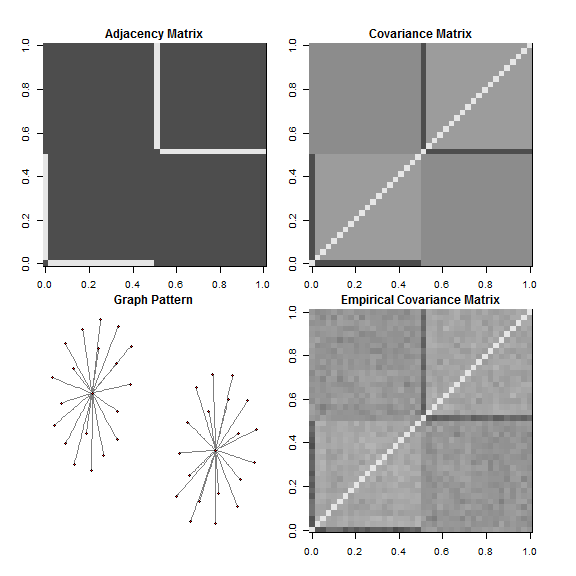``````plot(dat.cluster)
``````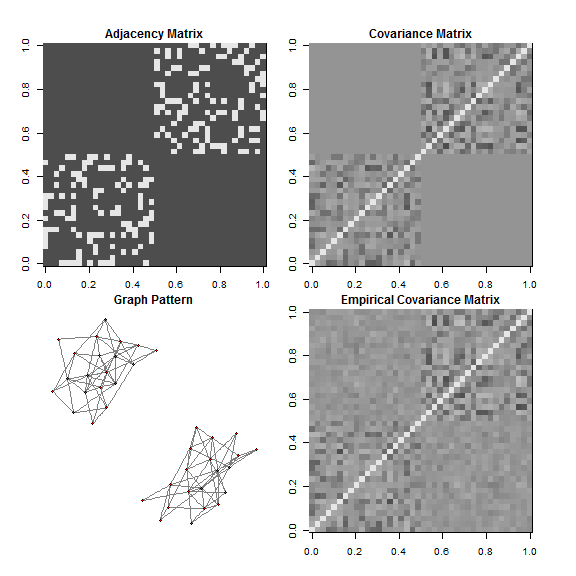``````plot(dat.rand)
``````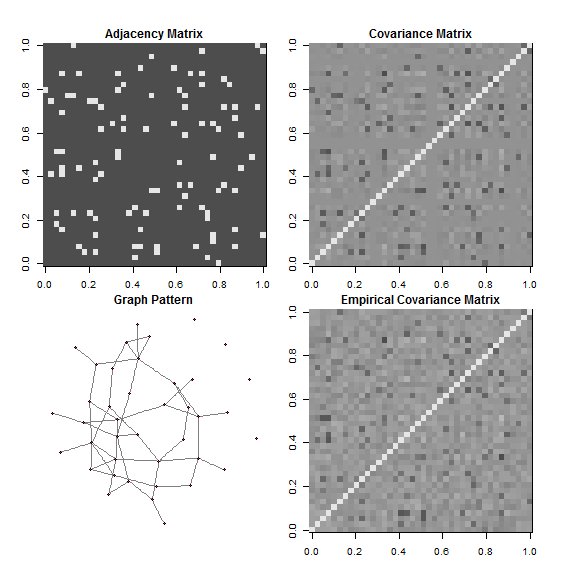# Stability selection

To run stability selection on a graphical model, we can simply use the dedicated `fitfun` called `quic.graphical_model`. Note that we don't supply a `y` argument to stabsel.

``````s.hubs <- stabsel(x = dat.hubs\$data, fitfun = quic.graphical_model,
cutoff = 0.75, PFER = 10)
s.cluster <- stabsel(x = dat.cluster\$data, fitfun = quic.graphical_model,
cutoff = 0.75, PFER = 10)
s.rand <- stabsel(x = dat.rand\$data, fitfun = quic.graphical_model,
cutoff = 0.75, PFER = 10)
``````

## Plot comparisons

In order to make comparisons possible, we use the following user defined plot function

``````plot_comparison <- function(stabsout, orig) {
## display comparison of original graph and stabs estimation
j <- orig\$omega * 0
orig.graph <- graph.adjacency(orig\$theta != 0, mode = "max", diag = FALSE)
ut <- upper.tri(j)
j[ut][stabsout\$selected] <- 1
stabs.graph <- graph.adjacency(j != 0, mode = "max", diag = FALSE)
layout <- layout.fruchterman.reingold(orig.graph)

par(mfrow = c(1,2))
plot(orig.graph, layout = layout, edge.color = "gray50", vertex.color = 'red',
main = "Real graph",  vertex.size = 3, vertex.label = NA)
plot(stabs.graph, layout = layout, edge.color = "gray50", vertex.color = 'red',
main = "Stabs estimated graph", vertex.size = 3, vertex.label = NA)
}
``````

Now, we compare the three graphs:

``````plot_comparison(s.hubs, dat.hubs)
``````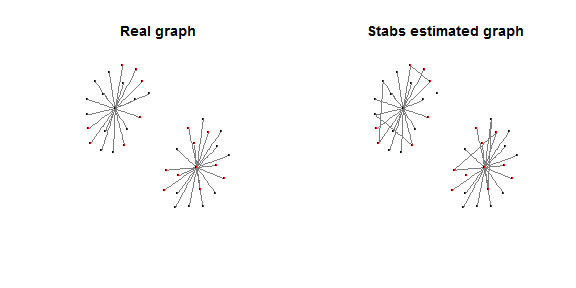``````plot_comparison(s.cluster, dat.cluster)
``````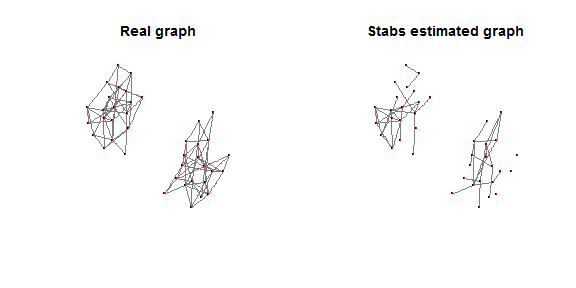``````plot_comparison(s.rand, dat.rand)
``````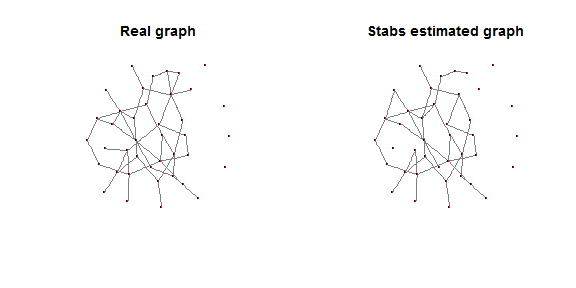As one can see, the original graphs are resembled by the estimated graphs rather well in this example.

# User specified `fitfun` for graphical models

For general instruction on how to create a `fitfun` please refer to

``````vignette("Using_stabs", package = "stabs")
``````

For an example for grahical model see

``````quic.graphical_model
``````
``````## function (x, y, q, ...)
## {
##     if (!requireNamespace("QUIC"))
##         stop("Package ", sQuote("QUIC"), " is required but not available")
##     extraargs <- list(...)
##     empirical.cov <- cov(x)
##     lams <- extraargs\$lams
##     if (is.null(lams)) {
##         max.cov <- max(abs(empirical.cov[upper.tri(empirical.cov)]))
##         lams <- getLamPath(max.cov, max.cov * 0.05, len = 40)
##     }
##     est <- QUIC::QUIC(empirical.cov, rho = 1, path = lams, msg = 0)
##     ut <- upper.tri(empirical.cov)
##     qvals <- sapply(1:length(lams), function(idx) {
##         m <- est\$X[, , idx]
##         sum(m[ut] != 0)
##     })
##     qq <- qvals >= q
##     if (!any(qq)) {
##         stop("Didn't reach the required number of variables. Try supplying lambda manually")
##     }
##     lamidx <- which.max(qvals >= q)
##     M <- est\$X[, , lamidx][ut]
##     selected <- (M != 0)
##     s <- sapply(1:lamidx, function(idx) {
##         m <- est\$X[, , idx][ut] != 0
##         return(m)
##     })
##     colnames(s) <- as.character(1:ncol(s))
##     return(list(selected = selected, path = s))
## }
## <bytecode: 0x00000000107a1970>
## <environment: namespace:stabs>
## attr(,"class")
##  "function"        "graphical_model"
``````

A speciality of graphical models is that the function has an additional class `"graphical_model"`. You can set this class immediately after having defined your `fitfun`, say, `my.graphical_model` as follows:

``````class(my.graphical_model) <- c(class(my.graphical_model), "graphical_model")
``````

This will avoid that stabsel expects a `y` argument and will change naming conventions for the resulting selections.